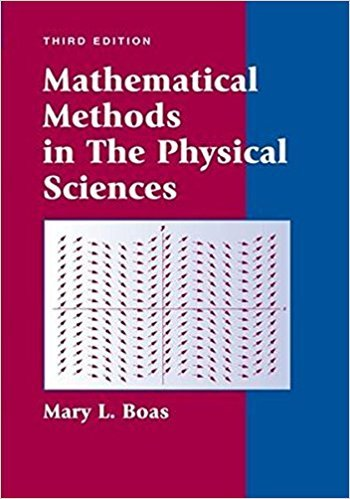×

# Solutions for Chapter 13: Partial Differential Equations## Full solutions for Mathematical Methods in the Physical Sciences | 3rd Edition

ISBN: 9780471198260Solutions for Chapter 13: Partial Differential Equations

Solutions for Chapter 13
4 5 0 359 Reviews
25
1
##### ISBN: 9780471198260

Chapter 13: Partial Differential Equations includes 28 full step-by-step solutions. Since 28 problems in chapter 13: Partial Differential Equations have been answered, more than 3583 students have viewed full step-by-step solutions from this chapter. This expansive textbook survival guide covers the following chapters and their solutions. This textbook survival guide was created for the textbook: Mathematical Methods in the Physical Sciences, edition: 3. Mathematical Methods in the Physical Sciences was written by and is associated to the ISBN: 9780471198260.

Key Physics Terms and definitions covered in this textbook
• //

parallel

• any symbol

average (indicated by a bar over a symbol—e.g., v¯ is average velocity)

• °C

Celsius degree

• °F

Fahrenheit degree

×